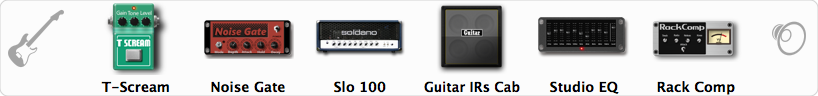# Dirty SLO100

Discussion in 'ToneLib-GFX presets' started by Diego Castro, May 17, 2019.

1. Dirty SLO100
GFX version: 3.0

Preset name: BasicMesaMetal
Optimized for: Guitar Amp

Effects chain:Good for Djent/Metal Volumes Style

Effect: "T-Scream" (Overdrive / Distortion), active - "yes"
"Drive" = 37
"Tone" = 100
"Level" = 79

Effect: "Noise Gate" (Dynamics / Filter), active - "yes"
"Detect" = Gtr In
"Mode" = Manual
"Depth" = 100
"Threshold" = 59
"Attack" = 0
"Hold" = 25
"Decay" = 5

Effect: "Slo 100" (Amp simulators), active - "yes"
"Gain" = 83
"Bass" = 62
"Middle" = 50
"Treble" = 50
"Presence" = 58
"Master" = 61
"Output" = 50
"Level (dB)" = 0

Effect: "Guitar IRs Cab" (Cabinets), active - "yes"
"Model" = Marshall 1960A (4x12")
"Mic Position" = Center
"Mic Distance" = Near
"Low Cut (Hz)" = 60
"Hi Cut (kHz)" = 20.0
"Mix" = 100
"Level (dB)" = 0

Effect: "Studio EQ" (Dynamics / Filter), active - "yes"
"31 Hz" = 0
"62 Hz" = 0
"125 Hz" = 0
"250 Hz" = -1
"500 Hz" = -1
"1 kHz" = 2
"2 kHz" = 0
"4 kHz" = 0
"8 kHz" = -2
"16 kHz" = -4
"above 16 kHz" = 0
"Level (dB)" = 0

Effect: "Rack Comp" (Dynamics / Filter), active - "yes"
"Threshold (dB)" = -6
"Ratio" = 5
"Attack" = Fast
"Release (ms)" = 250
"Knee" = 25
"Level (dB)" = 2

Note: This is a ToneLib-GFX preset file, you will need to download and install the ToneLib-GFX software to use the preset.

#### Attached Files:

• ###### Dirty_SOLO100.tlgfx
File size:
616 bytes
Views:
3,208
Last edited: May 17, 2019